Chapter 4 Appendix – Some practical issues

Review of Basic Electronics

A traditional presentation of this material might have begun with a review of the basic
concepts of direct current electricity.  Your author has elected to postpone that discussion
until this point in the course, at which time it is needed.

A Basic Circuit

We begin our discussion with a simple example circuit – a flashlight (or “electric torch” as
the Brits call it).  This has three basic components: a battery, a switch, and a light bulb.  For
our purpose, the flashlight has two possible states: on and off.  Here are two diagrams.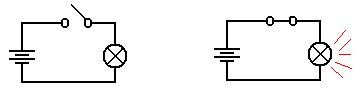Light is Off                              Light is On

In the both figures, we see a light bulb connected to a battery via two wires and a switch.
When the switch is open, it does not allow electricity to pass and the light is not illuminated.
When the switch is closed, the electronic circuit is completed and the light is illuminated.

The figure above uses a few of the following basic circuit elements.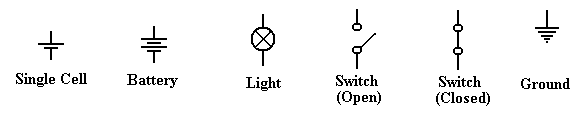We now describe each of these elements and then return to our flashlight example.  The first
thing we should do is be purists and note the difference between a cell and a battery, although
the distinction is quite irrelevant to this course.  A cell is what one buys in the stores today
and calls a battery; these come in various sizes, including AA, AAA, C, and D.  Each of
these cells is rated at 1.5 volts, due to a common technical basis for their manufacture.
Strictly speaking, a battery is a collection of cells, so that a typical flashlight contains one
battery that comprises two cells; usually AA, C, or D.  An automobile battery is truly a
battery, being built from a number of lead-acid cells.

A light is a device that converts electronic current into visible light.  This is not a surprise.
A switch is a mechanical device that is either open (not allowing transmission of current) or
closed (allowing the circuit to be completed).  Note that it is the opposite of a door, which
allows one to pass only when open.

The Idea of Ground

Consider the above circuit, which suggests a two-wire design: one wire from the battery to
the switch and then to the light bulb, and another wire from the bulb directly to the battery.
One should note that the circuit does not require two physical wires, only two distinct paths
for conducting electricity.  Consider the following possibility, in which the flashlight has a
metallic case that also conducts electricity.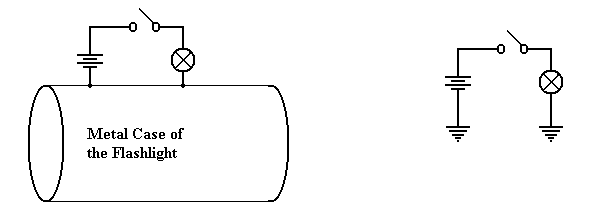Physical Connection                                                        Equivalent Circuit

Consider the circuit at left, which shows the physical connection postulated.  When the
switch is open, no current flows.  When the switch is closed, current flows from the battery
through the switch and light bulb, to the metallic case of the flashlight, which serves as a
return conduit to the battery.  Even if the metallic case is not a very good conductor, there
is much more of it and it will complete the circuit with no problem.

In electrical terms, the case of the battery is considered as a common ground, so that the
equivalent circuit is shown at right.  Note the new symbol in this circuit – this is the ground
element.  One can consider all ground elements to be connected by a wire, thus completing
the circuit.  In early days of radio, the ground was the metallic case of the radio – an
excellent conductor of electricity.  Modern automobiles use the metallic body of the car itself
as the ground.   Although iron and steel are not excellent conductors of electricity, the sheer
size of the car body allows for the electricity to flow easily.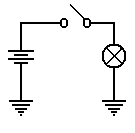To conclude, the circuit at left will be our representation of a
flashlight.  The battery provides the electricity, which flows through
the switch when the switch is closed, then through the light bulb, and
finally to the ground through which it returns to the battery.

As a convention, all switches in diagrams will be shown in the open
position unless there is a good reason not to.

The student should regard the above diagram as showing a switch which is not necessarily
open, but which might be closed in order to allow the flow of electricity.  The convention of
drawing a switch in the open position is due to the fact that it is easier to spot in a diagram.

Voltage, Current, and Resistance
It is now time to become a bit more precise in our discussion of electricity.  We need to
introduce a number of basic terms, many of which are named by analogy to flowing water.
The first term to define is current, usually denoted in equations by the symbol I.  We all
have an intuitive idea of what a current is.  Imagine standing on the bank of a river and
watching the water flow.  The faster the flow of water, the greater the current; flows of water
are often called currents.

In the electrical terms, current is the flow of electrons, which are one of the basic building
blocks of atoms.  While electrons are not the only basic particles that have charge, and are
not the only particle that can bear a current; they are the most common within the context of
electronic digital computers.  Were one interested in electro-chemistry he or she might be
more interested in the flow of positively charged ions.

All particles have one of three basic electronic charges: positive, negative, or neutral.  Within
an atom, the proton has the positive charge, the electron has the negative charge, and the
neutron has no charge.  In normal life, we do not see the interior of atoms, so our experience
with charges relates to electrons and ions.  A neutral atom is one that has the same number of
protons as it has electrons.  However, electrons can be quite mobile, so that an atom may gain
or lose electrons and, as a result, have too many electrons (becoming a negative ion) or too
few electrons (becoming a positive ion).  For the purposes of this course, we watch only the
electrons and ignore the ions.

An electric charge, usually denoted by the symbol Q, is usually associated with a large
number of electrons that are in excess of the number of positive ions available to balance
them.  The only way that an excess of electrons can be created is to move the electrons from
one region to another – robbing one region of electrons in order to give them to another.
This is exactly what a battery does – it is an electron “pump” that moves electrons from the
positive terminal to the negative terminal.  Absent any “pumping”, the electrons in the
negative terminal would return to the positive region, which is deficient in electrons, and
cause everything to become neutral.  But the pumping action of the battery prevents that.
Should one provide a conductive pathway between the positive and negative terminals of a
battery, the electrons will flow along that pathway, forming an electronic current.

To clarify the above description, we present the following diagram, which shows a battery, a
light bulb, and a closed switch.  We see that the flow of electrons within the battery is only a
part of a larger, complete circuit.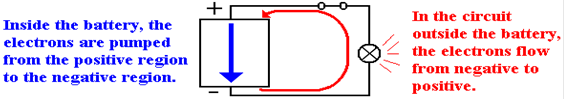Materials are often classified by their abilities to conduct electricity.  Here are two common
types of materials.

Conductor            A conductor is a substance, such as copper or silver, through which
electrons can flow fairly easily.

Insulator               An insulator is a substance, such as glass or wood, that offers
significant resistance to the flow of electrons.  In many of our
circuit diagrams we assume that insulators do not transmit electricity
at all, although they all do with some resistance.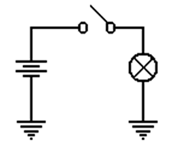The voltage is amount of pressure in the voltage pump.  It is quite
similar to water pressure in that it is the pressure on the electrons that
causes them to move through a conductor.  Consider again our
flashlight example.  The battery provides a pressure on the electrons
to cause them to flow through the circuit.  When the switch is open,
the flow is blocked and the electrons do not move.  When the switch
is closed, the electrons move in response to this pressure (voltage)
and flow through the light bulb.  The light bulb offers a specific
resistance to these electrons; it heats up and glows.

As mentioned above, different materials offer various abilities to transmit electric currents.
We have a term that measures the degree to which a material opposes the flow of electrons;
this is called resistance, denoted by R in most work.  Conductors have low resistance (often
approaching 0), while insulators have high resistance.  In resistors, the opposition to the flow
of electrons generates heat – this is the energy lost by the electrons as they flow through the
resistor.  In a light bulb, this heat causes the filament to become red hot and emit light.

An open switch can be considered as a circuit element of extremely high resistance.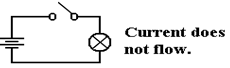Summary
We have discussed four terms so far.  We now should mention them again.

Charge           This refers to an unbalanced collection of electrons.  The term used
for denoting charge is Q.  The unit of charge is a coulomb.

Current          This refers to the rate at which a charge flows through a conductor.
The term used for denoting current is I.  The unit of current is an ampere.
Voltage           This refers to a force on the electrons that causes them to move.  This force
can be due to a number of causes – electro-chemical reactions in batteries
and changing magnetic fields in generators.  The term used for denoting
voltage is V or E (for Electromotive Force).  The unit of current is a volt.

Resistance      This is a measure of the degree to which a substance opposes the flow of
electrons.  The term for resistance is R.  The unit of resistance is an ohm.

Ohm’s Law and the Power Law
One way of stating Ohm’s law (named for Georg Simon Ohm, a German teacher who
discovered the law in 1827) is verbally as follows.

The current that flows through a circuit element is directly proportional to the
voltage across the circuit element and inversely proportional to the resistance
of that circuit element.

What that says is that doubling the voltage across a circuit element doubles the current flow
through the element, while doubling the resistance of the element halves the current.

Let’s look again at our flashlight example, this time with the switch shown as closed.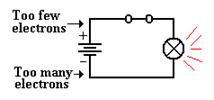The chemistry of the battery is pushing electrons away
from the positive terminal, denoted as “+” through the
battery towards the negative terminal, denoted as “–“.

This causes a voltage across the only resistive element
in the circuit – the light bulb.  This voltage placed across the light bulb causes current to
flow through it.

In algebraic terms, Ohm’s law is easily stated: E = I·R, where
E    is the voltage across the circuit element,
I     is the current through the circuit element, and
R   is the resistance of the circuit element.

Suppose that the light bulb has a resistance of 240 ohms and has a voltage of 120 volts
across it.  Then we say E = I·R or 120 = I·240 to get I = 0.5 amperes.

As noted above, an element resisting the flow of electrons absorbs energy from the flow it
obstructs and must emit that energy in some other form.  Power is the measure of the flow of
energy.  The power due to a resisting circuit element can easily be calculated.

The power law is states as P = E·I, where
P    is the power emitted by the circuit element, measured in watts,
E    is the voltage across the circuit element, and
I     is the current through the circuit element.

Thus a light bulb with a resistance of 240 ohms and a voltage of 120 volts across it has
a current of 0.5 amperes and a power of 0.5 · 120 = 60 watts.

There are a number of variants of the power law, based on substitutions from Ohm’s law.
Here are the three variants commonly seen.

P = E·I                        P = E2 / R                    P = I2·R

In our above example, we note that a voltage of 120 volts across a resistance of 60 ohms
would produce a power of P = (120)2 / 240 = 14400 / 240 = 60 watts, as expected.

The alert student will notice that the above power examples were based on AC circuit
elements, for which the idea of resistance and the associated power laws become more
complex (literally).  Except for a few cautionary notes, this course will completely ignore
the complexities of alternating current circuits.

Resistors in Series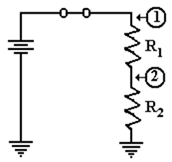There are very many interesting combinations of resistors found
in circuits, but here we focus on only one – resistors in series;
that is one resistor placed after another.  In this figure, we
introduce the symbol for a resistor.

Consider the circuit at right, with two resistors having
resistances of R1 and R2, respectively.  One of the basic laws of
electronics states that the resistance of the two in series is
simply the sum: thus R = R1 + R2.

Let E be the voltage provided by the battery.  Then the voltage across the pair of resistors is
given by E, and the current through the circuit elements is given by Ohm’s law as
I = E / (R1 + R2).  Note that we invoke another fundamental law
that the current through the two circuit elements in series must be the same.

Again applying Ohm’s law we can obtain the voltage drops across each of the two resistors.
Let E1 be the voltage drop across R1 and E2 be that across R2.  Then

E1 = I·R1 = R1·E / (R1 + R2), and
E2 = I·R2 = R2·E / (R1 + R2).
It should come as no surprise that E1 + E2      = R1·E / (R1 + R2) + R2·E / (R1 + R2)
= (R1 + R2)·E / (R1 + R2) = E.

If, as is commonly done, we assign the ground state as having zero voltage, then the voltages
at the two points in the circuit above are simple.

1)   At point 1, the voltage is E, the full voltage of the battery.
2)   At point 2, the voltage is E2 = I·R2 = R2·E / (R1 + R2).

Before we present the significance of the above circuit, consider two special cases.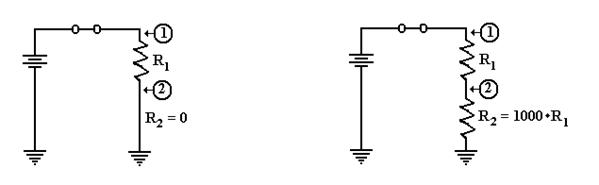In the circuit at left, the second resistor is replaced by a conductor having zero resistance.
The voltage at point 2 is then E2 = 0·E / (R1 + 0) = 0.  As point 2 is directly connected to
ground, we would expect it to be at zero voltage.

Suppose that R2 is much bigger than R1.  Let R1 = R and R2 = 1000·R.  We calculate the
voltage at point 2 as E2 = R2·E / (R1 + R2) = 1000·R·E / (R + 1000·R) = 1000·E/1001, or
approximately E2 = (1 – 1/1000)·E = 0.999·E.  Point 2 is essentially at full voltage.

Putting a Resistor and Switch in Series
We now consider an important circuit that is related to the above circuit.  In this circuit the
second resistor, R2, is replaced by a switch that can be either open or closed.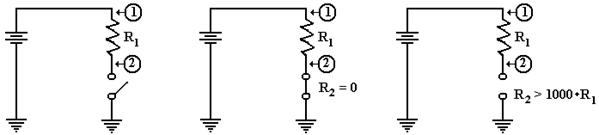The Circuit                            Switch Closed                          Switch Open

The circuit of interest is shown in the figure at left.  What we want to know is the voltage at
point 2 in the case that the switch is closed and in the case that the switch is open.  In both
cases the voltage at point 1 is the full voltage of the battery.

When the switch is closed, it becomes a resistor with no resistance; hence R2 = 0.  As we
noted above, this causes the voltage at point 2 to be equal to zero.

When the switch is open, it becomes equivalent to a very large resistor.  Some say that the
resistance of an open switch is infinite, as there is no path for the current to flow.  For our
purposes, it suffices to use the more precise idea that the resistance is very big, at least 1000
times the resistance of the first resistor, R1.  The voltage at point 2 is the full battery voltage.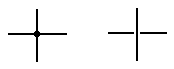Before we present our circuit, we introduce a notation used in
drawing two wires that appear to cross.  If a big dot is used at
the crossing, the two wires are connected.  If there is a gap, as in
the right figure, then the wires do not connect.

Here is a version of the circuit as we shall use it later.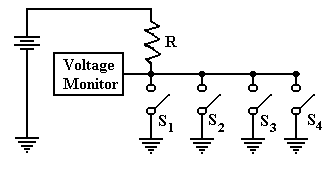In this circuit, there are four switches attached to the wire.  The voltage is monitored by
another circuit that is not important at this time.  If all four switches are open, then the
voltage monitor registers full voltage.  If one or more of the switches is closed, the monitor
registers zero voltage.  This is the best way to monitor a set of switches.

Back to Tri–State Buffers
We use the above verbiage to present a new view of tri–state buffers.  Consider the following
two circuits, which have been used previously in this chapter.  Suppose that the battery is
rated at five volts.  In the circuit at left, point A is at 5 volts and point B is at 0 volts.  In the
circuit at right, point B is clearly at 0 volts, but the status of point A is less clear.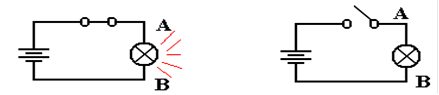What is obvious about the circuit at right is that there is no current flowing through it and no
power being emitted by the light bulb.  For this reason, we often say that point A is at 0 volts,
but it is better to say that there is no specified voltage at that point.  This is equivalent to the
third state of a tri–state buffer; the open switch is not asserting anything at point A.

Perhaps the major difference between the two circuits is that we can add another battery to
the circuit at right and define a different voltage at point A.  As long as the switch remains
open, we have no conflict.  Were the switch to be closed, we would have two circuits trying
to force a voltage at point A.  This could lead to a conflict.

Device Polling
Here is a more common use of tri–state buffers.  Suppose a number of devices, each of which
can signal a central voltage monitor by asserting logic zero (0 volts) on a line.  Recalling that
a logic AND outputs 0 if any of its inputs are 0, we could implement the circuit as follows.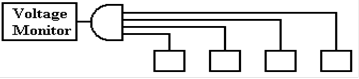Suppose we wanted to add another device.  This would require pulling the 4–input AND gate
and replacing it with a 5–input AND gate.  Continual addition of devices would push the
technology beyond the number of inputs a normal gate will support.

The tri–state solution avoids these problems.  This circuit repeats the one shown above with
the switches replaced by tri–state buffers, which should be viewed as switches.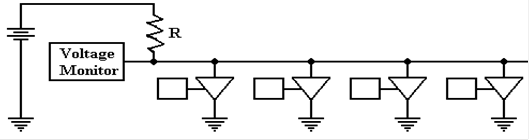One should note that additional devices can be added to this circuit merely by attaching
another tri–state switch.  The only limit to extensibility of this circuit arises from timing
considerations of signal propagation along the shared line.

Analysis of the Four–Tristate Circuit

In order to analyze the circuit at the bottom of the previous page, we refer back to the circuit
on the page before that.  We need to understand the voltage at the monitor, which is assumed
to be the input to a digital gate in the control logic of the CPU.  While a precise discussion of
this circuit involves treating resistors in parallel, such is not needed to be accurate here.

First, assume that none of the tri–states are enabled.  In that case, the circuit is equivalent to
the one in the next figure.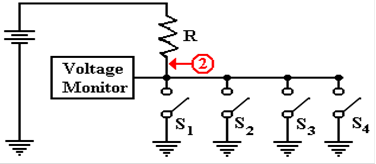The voltage at point 2 is the full
battery voltage, as the resistance
between that point and ground is
essentially infinite.

E2 = E / (1 + R1/R2)
E2 » E · (1 – R1/R2),
but R1/R2 » 0.

Now consider the situation in which one of the tri–state buffers is enabled.  Tri–state 2 has
been chosen arbitrarily.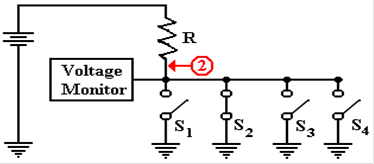Now there is a direct path of zero
resistance between point 2 and
ground.  The voltage at that point
drops to 0, with the entire
voltage drop being across
the resistor R.

Finally consider the situation in which more than one of the tri–state buffers is enabled.
As before, the choice is arbitrary.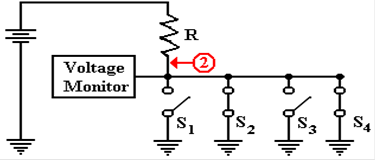Again, there is a direct path of
zero resistance between point 2
and ground.  The fact that there
are two such paths has no
practical consequences.  The
only criterion is one or more
path of zero resistance.

Multi–Media Logic

The purpose of this part of the appendix is for the student to become familiar with the
Multimedia logic emulation tool from Softronix, Inc.  The focus will be the following.

1.     Use of some of the tools; placing switches, basic components, and wires.

2.     Peculiarities of the switch control and the lack of a power supply.

The setup seems to be quite easy; I did not need to do anything but follow the directions.
(Here I am assuming that your computer is a standard one, running MS–Windows).

Start the tool.  The screen you see should resemble the following.This form follows the Microsoft standard, especially in the use of menus and tool bars.

While the tool can be used as is, there is a tool selection that will make it more intuitive.

Place the mouse cursor on the seventh tool from the left (Toggle Palette) and click it.
What one sees should resemble the following.Move the palette over to the right of the window to get the following.  I do not like to have
my controls placed on my work space.  Actually, you may place the control anywhere.Move the mouse cursor over the icons in the palette and identify the functions.  I get
Row 1:  Selector, Wire, Node
Row 2:  AND, OR, XOR

Find the switch control in the palette.  For me, it is the leftmost one on the fourth row.
Place a switch on the grid, towards the left part.  This is done by clicking on the control in the
palette and then clicking on a spot in the grid.  What you get may resemble the following.To move the switch, you must first click on the Selector tool (leftmost on the top row)
and then use the mouse cursor to drag the switch to its new position.

Now click on the LED control on the palette (rightmost on the third row) and place a
LED somewhere to the right of the switch.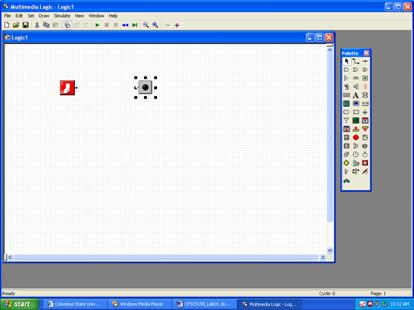It is now time to place a wire.  Select the Wire control on the palette (middle of the top row).
When you select this, each of the two circuit elements placed on the grid will display with a
single dot; the switch will have a dot to its right and the LED with a dot to its left.

After selecting the Wire control, click first on the dot for the switch and move the mouse to
the dot to the left of the LED, while holding down the mouse button.
You should see a wire drawn connecting the two.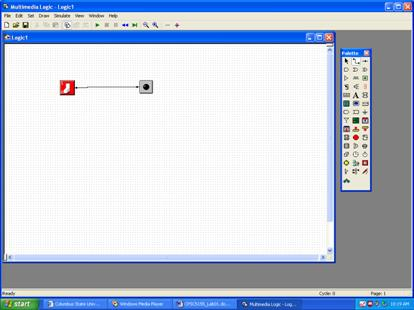Here, you see that I have drawn the wire “crooked”.  This is not a problem.  If you are a “neat
freak” like me, you can use the Selector tool to move either control to make the wire straight.

Go to the menu bar, select the Simulate menu tab, and then click on Run.  With the
simulation running, click on the switch.  The LED should turn on and off.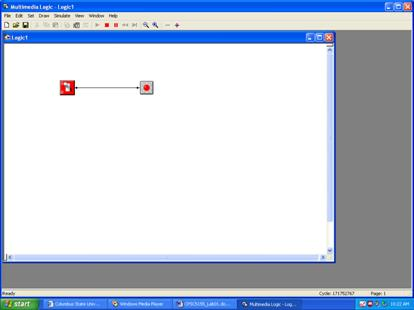Those of us who are more used to design of electrical circuits would find the above only
a partial circuit and expect to see something like the following.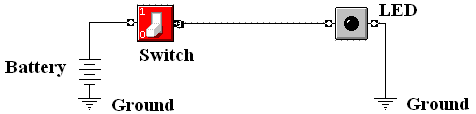This circuit shows the power supply explicitly, as well as the use of the ground connection to
complete the circuit.  Apparently, this tool supplies these features automatically.  Those of us
who do not work with circuits may feel free to ignore the figure above and just use the tool.

Next place two more switches, connect them to input of an AND gate, and connect the output
of the AND gate to a LED.  Run the simulation.  I get the following.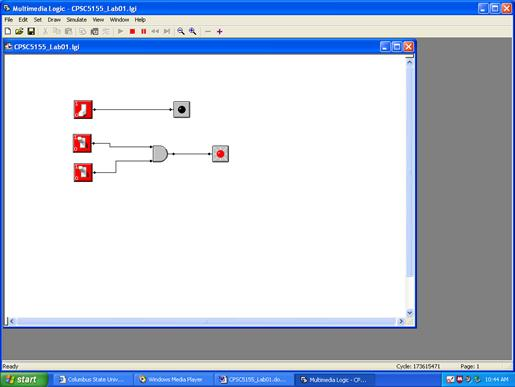This screen shot was taken with the simulator running, so that neither the grid nor the tool
palette is visible.  You should note that there are four possible combinations for the switch
positions: (OFF, OFF), (OFF, ON), (ON, OFF), and (ON, ON).  Record the status of the
LED for each of these four possibilities.

Finally add an OR gate (as shown below) and run the simulation again.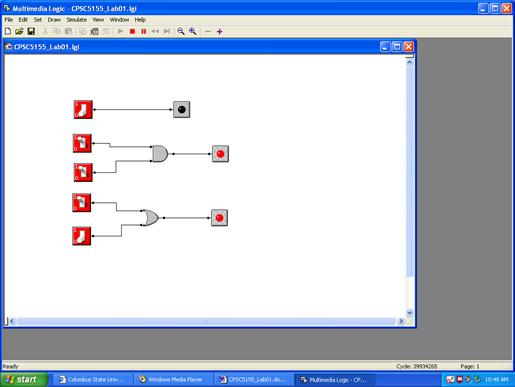Note that the OR outputs logic 1 when at least one of its inputs is set to logic 1 (the switch
is on).  You may want to play around with the NOT gate (Inverter) and XOR gate.

Some of you will note that it is possible to run the experiment above with only two switches.
More specifically, a switch can be used as input to more than one gate.  If you want to play